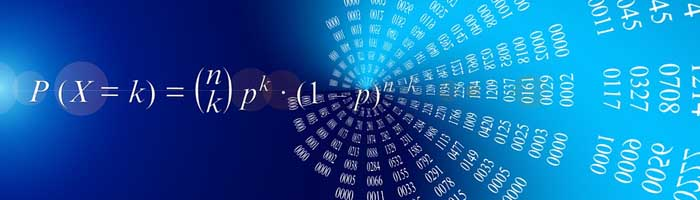Other Entrance Exams

IIT JAM Mathematics SyllabusIIT JAM Mathematics Syllabus

Maths Exam Pattern: Objective type, descriptive type and fill in the blanks questions

Marking Scheme: 1/4 mark will be deduct for wrong answer in objective type section.

Sequences and Series of real numbers:

Sequences and series of real numbers. Convergent and divergent sequences, bounded and monotone sequences, Convergence criteria for sequences of real numbers, Cauchy sequences, absolute and conditional convergence; Tests of convergence for series of positive terms – comparison test, ratio test, root test, Leibnitz test for convergence of alternating series.

Functions of one variable:

limit, continuity, differentiation, Rolle’s Theorem, Mean value theorem. Taylor’s theorem. Maxima and minima.

Functions of two real variable:

limit, continuity, partial derivatives, differentiability, maxima and minima.

Method of Lagrange multipliers, Homogeneous functions including Euler’s theorem.

Related: IIT JAM Entrance Exam Guide

Integral Calculus:

Integration as the inverse process of differentiation, definite integrals and their properties, Fundamental theorem of integral calculus. Double and triple integrals, change of order of integration. Calculating surface areas and volumes using double integrals and applications. Calculating volumes using triple integrals and applications.

Differential Equations:

Ordinary differential equations of the first order of the form y’=f(x,y). Bernoulli’s equation, exact differential equations, integrating factor, Orthogonal trajectories, Homogeneous differential equations-separable solutions, Linear differential equations of second and higher order with constant coefficients, method of variation of parameters. Cauchy- Euler equation.

Vector Calculus:

Scalar and vector fields, gradient, divergence, curl and Laplacian. Scalar line integrals and vector line integrals, scalar surface integrals and vector surface integrals, Green’s, Stokes and Gauss theorems and their applications.

Group Theory:

Groups, subgroups, Abelian groups, non-abelian groups, cyclic groups, permutation groups; Normal subgroups, Lagrange’s Theorem for finite groups, group homomorphisms and basic concepts of quotient groups (only group theory).

Linear Algebra:

Vector spaces, Linear dependence of vectors, basis, dimension, linear transformations, matrix representation with respect to an ordered basis, Range space and null space, rank-nullity theorem; Rank and inverse of a matrix, determinant, solutions of systems of linear equations, consistency conditions. Eigenvalues and eigenvectors. Cayley-Hamilton theorem. Symmetric, skew – symmetric, hermitian, skew-hermitian, orthogonal and unitary matrices.

Real Analysis:

Interior points, limit points, open sets, closed sets, bounded sets, connected sets, compact sets; completeness of R, Power series (of real variable) including Taylor’s and Maclaurin’s, domain of convergence, term-wise differentiation and integration of power series.